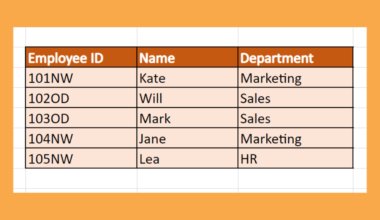# How to Set Decimal Places with Formula in Excel

We can use several Excel functions to set the number of decimal places with a formula.

This guide will be useful for situations where you want to modify a numerical value such that it has a certain number of decimal places.

Let’s take a look at a scenario where we can use Excel functions to set decimal places.

Suppose you have a table that computes the price of an item given the retail price and discount amount in percentages. Sometimes the result of this formula has more than two decimal places.

Since the US dollar only accounts for values with up to two decimal places, you would like to modify the result so that it becomes a valid price. How can we do this in Excel?

There are several functions in Excel that can set decimal places. First, we can use the `ROUND`, `ROUNDUP`, and `ROUNDDOWN` functions to calculate a rounded value from the original value. We can also use the `TRUNC` and `FLOOR` functions to provide a similar result.

Later in the article, we will discuss the differences between using each of these functions.

Now that we’ve described when you might need to set decimal places, we’ll look at a sample spreadsheet that uses each of these formulas.

## A Real Example of Setting Decimal Places with a Formula in Excel

Let’s take a look at a real example of the Excel functions being used in a spreadsheet.

In the example below, we have a list of contestants for a sprint event. Column B shows the recorded sprint time of each contestant. Suppose we wanted to only show two decimal places in the final tally. The table below shows the result of various methods to set the number of decimal places.First, we have the `ROUND` function. This function rounds a number to a specified number of digits. Each number can be rounded up or down depending on the value of the right-most digit included as a decimal place.

To get the values in Column C, we just need to use the following formula:

`=ROUND(B2,2)`

If you want the results to always be rounded up or rounded down, use the `ROUNDUP` or `ROUNDDOWN` function, respectively.

We can also use the `TRUNC` function to return a number with a specific number of decimal places. By default, `TRUNC` removes the fractional part of the number and returns an integer. However, we can use an optional argument to specify the precision of the truncation.

To get the values in Column F, we just need to use the following formula:

`=TRUNC(B2,2)`

Lastly, we can use the `FLOOR` function to set the number of decimal places. The `FLOOR` function rounds a number down to a given multiple. For example, `FLOOR(523.456,100)` would return 500 while `FLOOR(523.456, 0.01)` would return 523.45.

To get the values in Column F, we just need to use the following formula:

`=FLOOR(B2, 0.01)`

If you’re ready to try out these functions in Excel, let’s begin writing them ourselves!

## How to Set Decimal Places with Formula in Excel

This section will guide you through each step needed to set decimal places in Excel with various formulas.

1. First, we’ll show how to use the `ROUND` function to set the number of decimal places in Excel. Select the cell that will hold the result of our `ROUND` function.2. Next, type the equal sign ‘=‘ to begin the function, followed by ‘ROUND(‘.3. Add the original value as the first argument and indicate the number of decimal places as the second argument. In this example, our original value is located in cell B2 and we would like to keep only two decimal places. Hit the Enter key to evaluate the function.4. Fill in the rest of the values using AutoFill by dragging down the formula in the first cell.5. Instead of `ROUND`, we can also use `ROUNDUP`, `ROUNDDOWN`, `TRUNC`, and `FLOOR` to provide the necessary values.1. Can I use these techniques to convert values to integers?
Yes. You can use these same methods to convert your decimal values into an integer. For `ROUND`, `ROUNDDOWN`, `ROUNDUP`, and `TRUNC`, we can simply change the number of decimal places to 0. For the `FLOOR` function, we can set our second argument to 1 to return an integer.That’s all you need to remember to start using the `ROUND` function and others in Excel. This step-by-step guide explains how we can set decimal places in Excel easily.

The functions shown in this article are just some of the useful mathematical functions available in Excel. With so many other Excel functions out there, you can surely find some that you can take advantage of in your spreadsheets.

Are you interested in learning more about what Excel can do? Subscribe to our newsletter to be the first to know about the latest guides and tutorials from us.### Get emails from us about Excel.

Our goal this year is to create lots of rich, bite-sized tutorials for Excel users like you. If you liked this one, you'd love what we are working on! Readers receive ✨ early access ✨ to new content.

##### You May Also Like## How to Calculate a Cross Product in Excel

This guide will explain how to calculate a cross product in Excel. The cross product is a mathematical…## How to Use Wildcard in FILTER Function in Excel

This guide will explain how to use wildcards in the FILTER function in Excel. The rules for using…## How to Fix Formula Not Updating Automatically in Excel

This tutorial will explain a few ways to fix formulas not updating automatically in Microsoft Excel. By default,…## How to Calculate SMAPE in Excel

This guide will discuss how to calculate SMAPE in Excel. Since Excel contain several built-in functions and tools,…